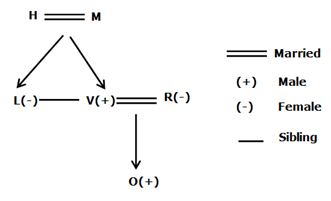# SBI Clerk Prelims Reasoning Ability Questions 2021 (Day-35)

Dear Aspirants, Our IBPS Guide team is providing new series of Reasoning Questions for SBI Clerk Prelims 2021 so the aspirants can practice it on a daily basis. These questions are framed by our skilled experts after understanding your needs thoroughly. Aspirants can practice these new series questions daily to familiarize with the exact exam pattern and make your preparation effective.

Start Quiz

Unknown based linear

Direction (1-5): Study the following information carefully and answer the below questions.

A certain number of persons are sitting in a row facing north. Three persons are sitting between M and G. L sits second to the right of R. T sits third to the left of M. O sits exactly between G and R. X sits at the end of the row. The number of seats between G and M is the same as between G and O. Not more than two persons are sitting between X and T. The number of persons sitting to the left of T is one more than the right of R.

1) What is the position of T with respect to O?

A.Ninth to the right

B.Eighth to the left

C.Tenth to the left

D.Eleventh to the left

E.Fifth to the right

2) How many persons are sitting in the row?

A.22

B.21

C.17

D.20

E.19

3) Four of the following five are similar in such a way that they form a group, who among the following doesn’t belong to the group?

A.M

B.G

C.the one who sits third to the right of X

D.O

E.the one who sits second to the right of R

4) Who among the following person sits sixth to the left of G?

A.the one who sits third to the right of X

B.None of these

C.M

D.O

E.the one who sits second to the right of R

5) Which of the following statement is/are false?

I). Only five seats are between M and X

II). T sits exactly between M and X

III). More than six persons are sitting to the right of O.

A.Both I and III

B.Only III

C.Both I and II

D.Only II

E.None of the statement is false

Direction sense

Direction (6-8): Study the following information carefully and answer the below questions.

Point A is 5km south of Point D which is 8km west of Point F. Point C is 4km west of Point P which is 5km north of Point N. Point H is 10km south of point F and 16km east of point N.

6) What is the direction of Point D with respect to Point H?

A.East

B.West

C.North-west

D.South

E.South-west

7) If Point T is exactly between the points F and H then what is the distance between points T and C?

A.18km

B.14km

C.12km

D.16km

E.20km

8) Which of the following point is not to the south-west of point D?

A.C

B.P

C.N

D.H

E.All the above

Blood rleation

Direction (9-10): Study the following information carefully and answer the below questions.

A family consists of six members. H is the spouse of M who has two children. M is the grandparent of O who is the nephew of L. R is the sister-in-law of L who is of opposite gender of V. L is an unmarried member.

9) How is H related to V?

A.Mother

B.Sister

C.Father

D.Brother

E.Can’t be determined

10) Who among the following person is the spouse of R?

A.V

B.The one who is the Father of O

C.The one who is the Brother of L

D.The one who is the Son of H

E.All of the above

Directions (1-5) :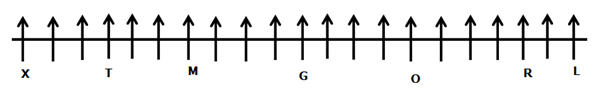Three persons are sitting between M and G.

T sits third to the left of M.

From the above condition, there are two possibilities.The number of seats between G and M is the same as between G and O.

O sits exactly between G and R.

L sits second to the right of R.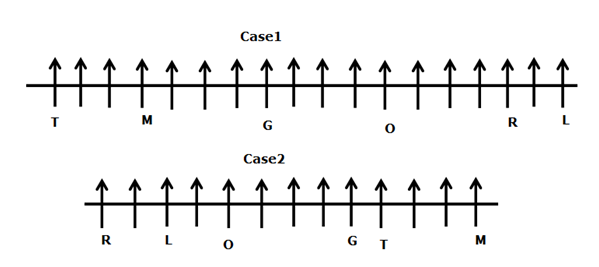The number of persons sitting to the left of T is one more than the right of R.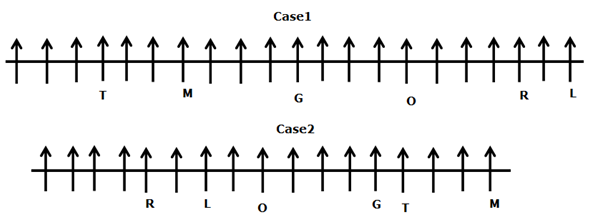X sits at the end of the row.

Not more than two persons are sitting between X and T.

From the above condition, case2 gets eliminated. case1 shows the final arrangement.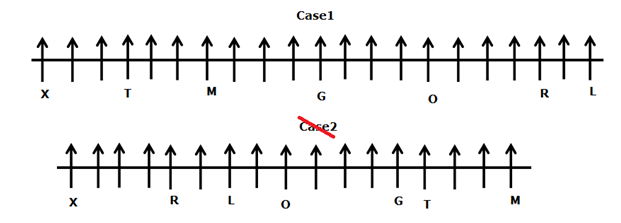Directions (6-8):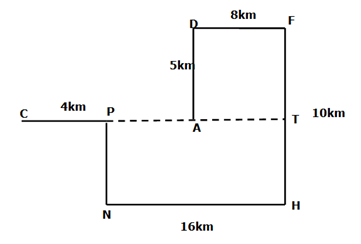Directions (9-10):